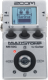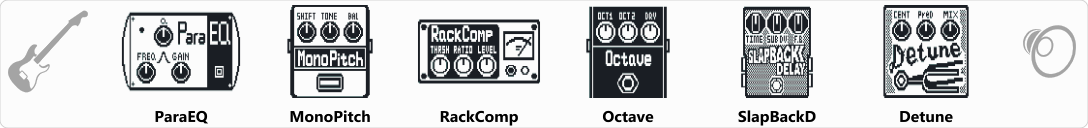# Chapman Stick

Discussion in 'Zoom MS-50G' started by John Cope, Jun 15, 2022.

1. Chapman StickDevice: Zoom Ms-50g
Firmware: 3.10

Name on device: Chapman
Optimized for: Guitar Amp

Effects chain:Chapman Stick Bass effect. Emulates Tony Levin's Funkfingers

Effect: "ParaEQ" (Dynamics / Filter), active - "yes"
"Freq1" = 400Hz
"Q1" = 16
"Gain1" = 12
"Freq2" = 250Hz
"Q2" = 8
"Gain2" = 11
"Level" = 150

Effect: "MonoPitch" (Modulation / Sfx), active - "yes"
"Shift" = 0
"Tone" = 10
"Bal" = 50
"Fine" = -9
"Level" = 132

Effect: "RackComp" (Dynamics / Filter), active - "yes"
"THRSH" = 41
"Ratio" = 8
"Level" = 133
"ATTCK" = 10

Effect: "Octave" (Modulation / Sfx), active - "yes"
"Oct1" = 19
"Oct2" = 21
"Dry" = 99
"Chara" = 56
"Tone" = 7
"Level" = 94

Effect: "SlapBackD" (Delay / Reverb), active - "yes"
"TIME" = 12
"SubDv" = 1/4
"F.B" = 34
"FxLVL" = 40
"DRY" = On
"Tail" = On
"Mode" = On

Effect: "Detune" (Modulation / Sfx), active - "yes"
"Cent" = 10
"PreD" = 14
"Mix" = 52
"Tone" = 8
"Level" = 100

Note: This is a patch file, you will need to download and install the ToneLib-Zoom software to use the patch.

File size:
437 bytes
Views:
61